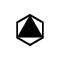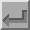Sixfold Inversion Axis (-6)The symbol above shows a sixfold inversion axis, i.e. a sixfold inversion axis perpendicular to the screen. A fractional value next to the symbol indicates the height of the inversion centre above the XY plane. As with the sixfold axis, a positive rotation is anticlockwise. A sixfold rotation about the c-axis, i.e. about the line 0,0,z, followed by an inversion at the origin will have the corresponding symmetry operator -x+y,-x,-z. This axis has the written symbol "-6" (where the minus sign is usually written above the digit). The solid triangle at the centre of the symbol emphasises the implied threefold axis that results from a sixfold inversion axis.Back
 © Copyright 1995-2006.  Birkbeck College, University of London. Author(s): Jeremy Karl Cockcroft